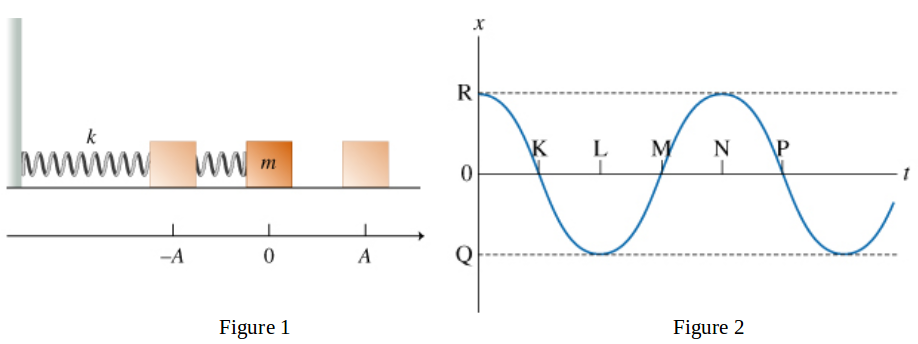# Problem: Introduction to OscillationsThe conditions that lead to simple harmonic motion are as follows:There must be a position of stable equilibrium.There must be a restoring force acting on the oscillating object. The direction of this force must always point toward the equilibrium, and its magnitude must be directly proportional to the magnitude of the object's displacement from its equilibrium position. Mathematically, the restoring force F⃗  is given by F⃗ = −kx⃗, where x⃗  is the displacement from equilibrium and k is a constant that depends on the properties of the oscillating system.The resistive forces in the system must be reasonably small.Consider a block of mass m attached to a spring with force constant k, as shown in the figure (Figure 1). The spring can be either stretched or compressed. The block slides on a frictionless horizontal surface, as shown. When the spring is relaxed, the block is located at x=0. If the block is pulled to the right a distance A and then released, A will be the amplitude of the resulting oscillations.Assume that the mechanical energy of the block-spring system remains unchanged in the subsequent motion of the block.Now assume for the remaining Parts G -J, that the x coordinate of point R is 0.12 m and the t coordinate of point K is 0.0050 s.What distance d does the object cover during one period of oscillation? Express your answer in meters.

###### FREE Expert Solution

d = 4A

98% (334 ratings)###### Problem Details

Introduction to Oscillations

The conditions that lead to simple harmonic motion are as follows:

1. There must be a position of stable equilibrium.
2. There must be a restoring force acting on the oscillating object. The direction of this force must always point toward the equilibrium, and its magnitude must be directly proportional to the magnitude of the object's displacement from its equilibrium position. Mathematically, the restoring force F⃗  is given by F⃗ = −kx⃗, where x⃗  is the displacement from equilibrium and k is a constant that depends on the properties of the oscillating system.
3. The resistive forces in the system must be reasonably small.

Consider a block of mass m attached to a spring with force constant k, as shown in the figure (Figure 1). The spring can be either stretched or compressed. The block slides on a frictionless horizontal surface, as shown. When the spring is relaxed, the block is located at x=0. If the block is pulled to the right a distance A and then released, A will be the amplitude of the resulting oscillations.

Assume that the mechanical energy of the block-spring system remains unchanged in the subsequent motion of the block.Now assume for the remaining Parts G -J, that the x coordinate of point R is 0.12 m and the t coordinate of point K is 0.0050 s.

What distance d does the object cover during one period of oscillation? Express your answer in meters.